# Korycansky and Papaloizou (1996)

Korycansky & Papaloizou (1996, ApJS, 105, 181; hereafter KP96) developed a method to find nontrivial, nonaxisymmetric steady-state flows in a two-dimensional setting. Specifically, they constructed infinitesimally thin steady-state disk structures in the presence of a time-independent, nonaxisymmetric perturbing potential. While their problem was only two-dimensional and they did not seek a self-consistent solution of the gravitational Poisson equation, the approach they took to solving the 2D Euler equation in tandem with the continuity equation for a compressible fluid is instructive. What follows is a summary of their approach.

KP96 begin with the standard set of principal governing equations, but choose to work from the set that is expressed in terms of a rotating frame of reference. (Throughout the presentation on this page, it is to be understood that all variables are viewed from a rotating frame even though the subscript notation "rot" does not appear in the equations.) Their Eq. (1), for example, comes from the

Eulerian Representation
of the Continuity Equation
as viewed from a Rotating Reference Frame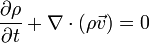$\frac{\partial\rho}{\partial t} + \nabla \cdot (\rho {\vec{v}}) = 0$ ,

and the,

Eulerian Representation
of the Euler Equation
as viewed from a Rotating Reference Frame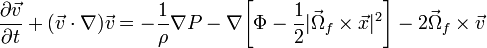$\frac{\partial\vec{v}}{\partial t} + ({\vec{v}}\cdot \nabla) {\vec{v}} = - \frac{1}{\rho} \nabla P - \nabla \biggl[\Phi - \frac{1}{2}|{\vec{\Omega}}_f \times \vec{x}|^2 \biggr] - 2{\vec{\Omega}}_f \times {\vec{v}}$ .

And their Eq. (7) can be derived straightforwardly from the

Euler Equation
written in terms of the Vorticity and
as viewed from a Rotating Reference Frame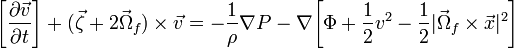$\biggl[\frac{\partial\vec{v}}{\partial t}\biggr] + ({\vec{\zeta}}+2{\vec\Omega}_f) \times {\vec{v}}= - \frac{1}{\rho} \nabla P - \nabla \biggl[\Phi + \frac{1}{2}v^2 - \frac{1}{2}|{\vec{\Omega}}_f \times \vec{x}|^2 \biggr]$ .

Assumption #1: KP96 align the angular velocity vector of the rotating frame of reference with the z-axis of a Cartesian coordinate system. Specifically, they set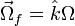${\vec{\Omega}}_f = \hat{k}\Omega$.

Assumption #2:Because KP96 are seeking steady-state solutions, they set all Eulerian time-derivatives to zero.

Hence, the steady-state versions of the Euler and continuity equations shown above give rise to Eq. (1) of KP96, namely,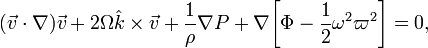$(\vec{v}\cdot \nabla)\vec{v} + 2\Omega\hat{k}\times\vec{v} + \frac{1}{\rho}\nabla P + \nabla \biggl[\Phi -\frac{1}{2}\omega^2 \varpi^2 \biggr] = 0 ,$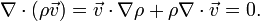$\nabla\cdot(\rho \vec{v}) = \vec{v}\cdot\nabla\rho + \rho\nabla\cdot\vec{v} = 0 .$

And, if written in terms of the vorticity, our steady-state Euler equation becomes essentially Eq. (7) of KP96, namely,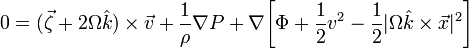$0 = ({\vec{\zeta}}+2\Omega{\hat{k}}) \times {\vec{v}} + \frac{1}{\rho} \nabla P + \nabla \biggl[\Phi + \frac{1}{2}v^2 - \frac{1}{2}|\Omega\hat{k} \times \vec{x}|^2 \biggr]$$= - {\vec{v}}\times({\vec{\zeta}}+2\Omega{\hat{k}}) + \nabla \biggl[\frac{1}{2}v^2 + H + \Phi - \frac{1}{2}|\Omega\hat{k} \times \vec{x}|^2 \biggr] ,$

where, in this last expression, we have replaced the gradient of the pressure by the gradient of the enthalpy via the relation,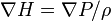$\nabla H = \nabla P/\rho$. Note that the KP96 notation is slightly different from ours:

• Σ is used in place of ρ to denote a two-dimensional surface density;
•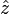$\hat{z}$ is used instead of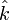$\hat{k}$ to denote a unit vector in the z-coordinate direction;
• the vorticity vector is written as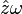$\hat{z}\omega$ instead of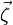$\vec\zeta$;
• W is used instead of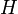$~H$ to denote the enthalpy; and
• Φg represents the combined, time-independent gravitational and centrifugal potential, that is,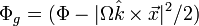$\Phi_g = (\Phi - |\Omega\hat{k} \times \vec{x}|^2/2)$.

Up to this point, only the two assumptions itemized above have been imposed on the key governing equations. Hence, although KP96 apply these equations to the study of a two-dimensional flow problem, our derived forms for the equations can serve to describe a fully 3D problem.

Staying with this generalized approach, let's examine a few more aspects of these governing relations before focusing in on the more restrictive, 2D problem that has been tackled in KP96. First, let's rewrite the steady-state Euler equation in the form,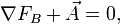$\nabla F_B + \vec{A} = 0 ,$

where, the scalar "Bernoulli" function,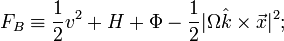$F_B \equiv \frac{1}{2}v^2 + H + \Phi - \frac{1}{2}|\Omega\hat{k} \times \vec{x}|^2 ;$

and,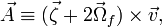$\vec{A} \equiv ({\vec{\zeta}}+2{\vec\Omega}_f) \times {\vec{v}} ,$

is the vector involving a nonlinear cross-product of the velocity that has been introduced in our accompanying general discussion of the Euler equation as viewed from a rotating frame of reference.

### Scalar Product of Velocity and Euler

If we dot the vector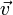$\vec{v}$ into the steady-state Euler equation, we obtain the expression,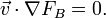$\vec{v} \cdot \nabla F_B = 0 .$

The vector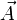$\vec{A}$ disappears as a result of the dot product with$\vec{v}$ because$\vec{A}$ is necessarily everywhere perpendicular to$\vec{v}$.

### Curl of Euler

If, on the other hand, we take the curl of the steady-state Euler equation, we obtain the expression,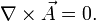$\nabla\times\vec{A} = 0 .$

In this case the gradient of the Bernoulli function disappears because the curl of any gradient is zero. This vector equation provides three independent physical constraints on our system, as all three Cartesian components of the curl of$\vec{A}$ must independently be zero. Expressions for the three components of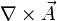$\nabla\times\vec{A}$ can be found in our accompanying general discussion of the Euler equation as viewed from a rotating frame of reference.

## Two-Dimensional Planar Flow

In keeping with their objective to study steady-state flows in infinitesimally thin disks, KP96 imposed one additional important constraint on the set of governing equations.

Assumption #3: KP96 set vz = 0 everywhere.

Then, in order to determine the steady-state spatial distribution of the three principal physical variables ρ(x,y), vx(x,y), and vy(x,y), they looked for solutions that would simultaneously satisfy the following three PDEs:

• The z-component of the curl of the steady-state Euler equation, that is,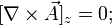$[\nabla\times\vec{A}]_z = 0 ;$

• The steady-state continuity equation, that is,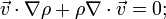$\vec{v}\cdot\nabla\rho + \rho\nabla\cdot\vec{v} = 0 ;$

and,

• The scalar product of$\vec{v}$ with the steady-state Euler equation, that is,$\vec{v} \cdot \nabla F_B = 0 .$

The algebraic equation of state that they used to supplement this coupled set of governing PDEs is identified in their paper, in the discussion associated with their Eq. (23).

Drawing from our accompanying discussion of how the curl of$\vec{A}$ behaves when vz = 0, the first of these PDEs takes the form,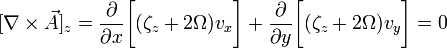$[\nabla\times\vec{A}]_z = \frac{\partial}{\partial x}\biggl[ (\zeta_z + 2\Omega) v_x \biggr] + \frac{\partial}{\partial y}\biggl[ (\zeta_z + 2\Omega) v_y \biggr] = 0$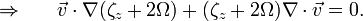$\Rightarrow ~~~~~\vec{v}\cdot\nabla(\zeta_z + 2\Omega) + (\zeta_z + 2\Omega)\nabla\cdot\vec{v} = 0 .$

This last expression appears as Eq. (2) in KP96. (For this last expression to be valid it must be understood that, for the inherently 2D problem under investigation by KP96,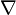$\nabla$ is only operating in x and y.) Rewriting this equation, we conclude that,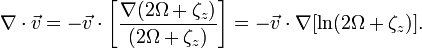$\nabla\cdot\vec{v} =-\vec{v} \cdot \biggl[ \frac{\nabla(2\Omega + \zeta_z)}{(2\Omega + \zeta_z)} \biggr] = -\vec{v} \cdot \nabla[\ln(2\Omega + \zeta_z)].$

But from the steady-state continuity equation we also know that,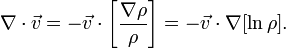$\nabla\cdot\vec{v} = -\vec{v}\cdot\biggl[\frac{\nabla\rho}{\rho} \biggr] = -\vec{v} \cdot \nabla[\ln\rho] .$

Hence, combining the two relations, we conclude that,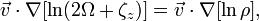$\vec{v} \cdot \nabla[\ln(2\Omega + \zeta_z)] = \vec{v} \cdot \nabla[\ln\rho] ,$

that is,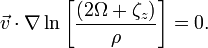$\vec{v} \cdot \nabla\ln\biggl[ \frac{(2\Omega + \zeta_z)}{\rho} \biggr] = 0 .$

This is essentially KP96's Eq. (3). It tells us that, in the steady-state flow whose spatial structure we are seeking, the velocity vector (and also the momentum density vector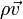$\rho\vec{v}$) must everywhere be tangent to contours of constant (2Ω + ζz) / ρ — a scalar quantity that KP96 refer to as vortensity.

Introduce stream function: The constraint implied by the continuity equation also suggests that it might be useful to define a stream function in terms of the momentum density — instead of in terms of just the velocity, which is the natural treatment in the context of incompressible fluid flows. KP96 do this. They define the stream function, Ψ, such that (see their Eq. 4),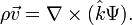$\rho\vec{v} = \nabla\times(\hat{k}\Psi) .$

in which case,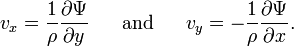$v_x = \frac{1}{\rho} \frac{\partial \Psi}{\partial y} ~~~~~\mathrm{and}~~~~~ v_y = - \frac{1}{\rho} \frac{\partial \Psi}{\partial x} .$

This implies as well that the z-component of the fluid vorticity can be expressed in terms of the stream function as follows (see Eq. 5 of KP96):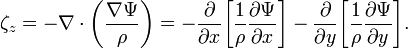$\zeta_z = - \nabla\cdot \biggl( \frac{\nabla\Psi}{\rho} \biggr) = - \frac{\partial}{\partial x} \biggl[ \frac{1}{\rho} \frac{\partial\Psi}{\partial x} \biggr] - \frac{\partial}{\partial y} \biggl[ \frac{1}{\rho} \frac{\partial\Psi}{\partial y} \biggr].$

Since, by design, streamlines defined by the momentum-density vector field must trace out lines of constant Ψ and, according to the conclusion drawn above, the momentum density vector must everywhere be tangent to contours of constant vortensity, we can conclude — as did KP96 — that the vortensity z + 2Ω) / ρ must be constant along streamlines. The vortensity is therefore a function of Ψ alone, so we can write,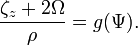$\frac{\zeta_z + 2\Omega}{\rho} = g(\Psi) .$

By the same token, the condition obtained from the scalar product of$\vec{v}$ with the steady-state Euler equation,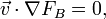$\vec{v} \cdot \nabla F_B = 0 ,$

implies that the Bernoulli function must also be expressible as a function of Ψ alone. Hence, we can write,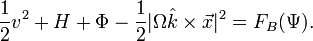$\frac{1}{2}v^2 + H + \Phi - \frac{1}{2}|\Omega\hat{k} \times \vec{x}|^2 = F_B(\Psi) .$

KP96 then go on to demonstrate that the relationship between the functions g(Ψ) and F(Ψ) is,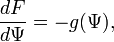$\frac{dF}{d\Psi} = -g(\Psi) ,$

which allows the determination of F up to a constant of integration.

## Summary

In summary, KP96 constrain their flow as follows:

1. They use the z-component of the curl of the Euler equation;
2. They use the compressible version of the continuity equation;
3. Instead of taking the divergence of the Euler equation to obtain a Poisson-like equation, they obtain an algebraic constraint on the Bernoulli function (as in our traditional SCF technique) by simply "dotting"$\vec{v}$ into the Euler equation.

# Suggested Strategy by Joel

For the time being, I want to continue to discuss only planar flows. Aside from the Poisson equation and the equation of state, the three key scalar equations are:

1. Continuity equation;
2. Z-component of the curl of the Euler equation; and
3. Scalar product of$\vec{v}$ and Euler.

Let's take one of Shangli's compressible Riemann ellipsoids as our starting guess. We need to "tilt" each of the velocity vectors slightly so that they everywhere satisfy the compressible equation of state. By combining the first two of the three key scalar equations, the KP96 study tells us that we can do this not by orienting them tangent to isodensity contours but, rather, by orienting them tangent to iso-vortensity surfaces. That is, we need,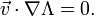$\vec{v} \cdot \nabla\Lambda = 0 .$

where,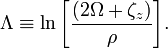$\Lambda \equiv \ln\biggl[ \frac{(2\Omega + \zeta_z)}{\rho} \biggr] .$

Let's rely on this expression only to tell us how to orient each velocity vector, that is only to give us the ratio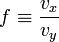$f \equiv \frac{v_x}{v_y}$

everywhere. And let's assume that Ω,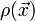$\rho(\vec{x})$ and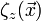$\zeta_z(\vec{x})$ are known from Shangli's model. Then we can straightforwardly conclude that, at all spatial locations within the configuration,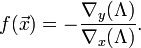$f(\vec{x}) = -\frac{\nabla_y(\Lambda)}{\nabla_x(\Lambda)} .$

Everywhere along the surface,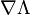$\nabla\Lambda$ may not be well-determined; in which case I suspect that we should align the velocity vectors tangent to the surface.

Next, let's use either the continuity equation or the z-component of the curl of Euler to determine the updated magnitude,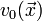$v_0(\vec{x})$, of each velocity vector. Using the continuity equation, for example, we need,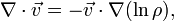$\nabla\cdot\vec{v} = -\vec{v}\cdot\nabla(\ln\rho) ,$

where, in terms of the function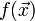$f(\vec{x})$,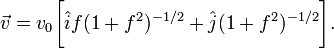$\vec{v} = v_0 \biggl[ \hat{i} f ( 1 + f^2 )^{-1/2} + \hat{j} ( 1 + f^2 )^{-1/2} \biggr] .$

Let me emphasize that, during this step of our SCF iteration cycle, we should assume that the functions$\rho(\vec{x})$ and$f(\vec{x})$ are known throughout the configuration. The continuity equation can therefore be written as,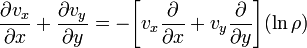$\frac{\partial v_x}{\partial x} + \frac{\partial v_y}{\partial y} = - \biggl[ v_x\frac{\partial}{\partial x} + v_y \frac{\partial}{\partial y} \biggr] (\ln\rho)$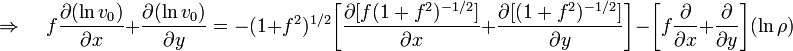$\Rightarrow ~~~~~ f \frac{\partial (\ln v_0)}{\partial x} + \frac{\partial (\ln v_0)}{\partial y} = - ( 1 + f^2 )^{1/2} \biggl[ \frac{\partial [f ( 1 + f^2 )^{-1/2}]}{\partial x} + \frac{\partial [( 1 + f^2 )^{-1/2}]}{\partial y} \biggr] - \biggl[ f \frac{\partial}{\partial x} + \frac{\partial}{\partial y} \biggr] (\ln\rho)$

 © 2014 - 2020 by Joel E. Tohline |   H_Book Home   |   YouTube   | Appendices: | Equations | Variables | References | Ramblings | Images | myphys.lsu | ADS |